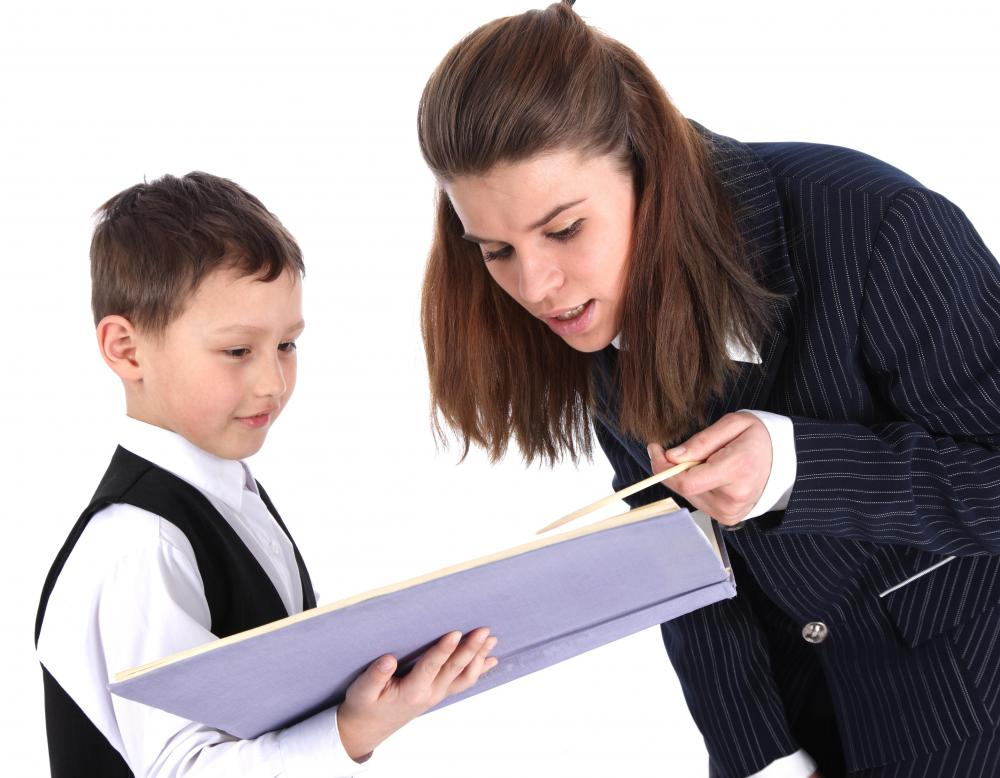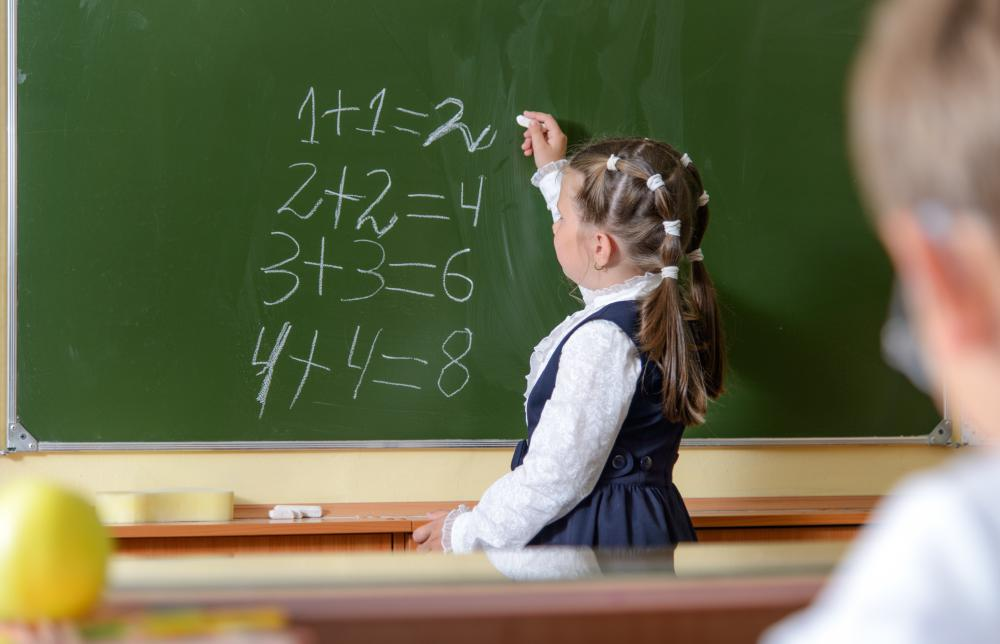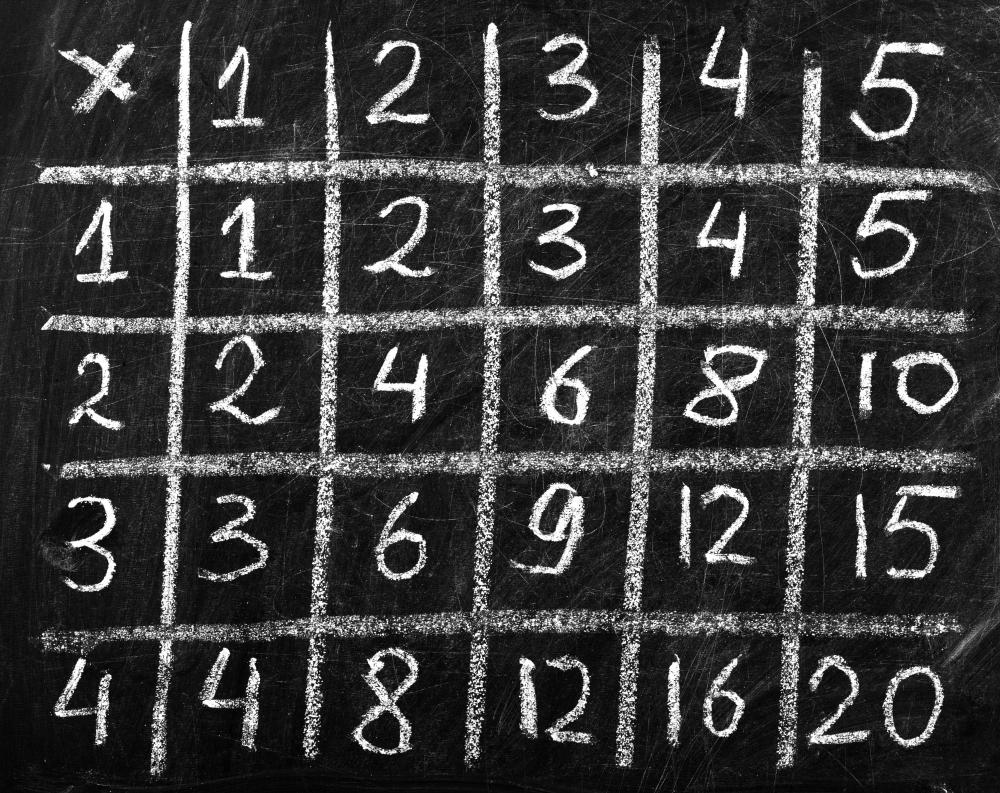# What Are the Best Tips for Teaching Children Mathematics?

Dee Jones

There is no underestimating the importance of teaching children mathematics. Children who are able to understand the basic mathematical concepts are much more likely to excel in school. Teaching children mathematics is a progressive process. One should start by teaching the child the basics of addition, followed by subtraction and multiplication, then ending with division, which is often the hardest mathematical concept for a child to learn.

When teaching children mathematics, teaching them addition is the first step in giving them a strong mathematical foundation, which will make learning subtraction, multiplication and division easier. Fortunately, addition is one of the simplest mathematical concepts, and teaching a child the basics of doing simple addition just takes a little time, patience and persistence. The simplest way to start is by teaching the child to add together groups of tangible objects, such as pennies, jelly beans or pebbles. For example, a teacher or parent might place four pennies in one pile and six pennies in another pile, then explain to the child that these two piles represent the addition problem 4 + 6. When the child adds the pennies together and concludes that there are 10 pennies in all, it can be explained that this means that 4 + 6 = 10.Children who understand basic mathematical concepts are much more likely to excel in school.

After learning the basics of addition, the next step to teaching kids mathematics is helping them to understand the basic principles of subtraction. As with addition, using tangible objects will make it easier for a child to understand the basic concepts. It will also help them grasp that subtraction is really just addition in reverse. For example, one could start with a pile of 10 jelly beans and allow the child to count them. The teacher can explain to the child that three jelly beans are going to be removed from the pile, which will represent the subtraction problem 10 - 3, and the jelly beans left in the pile will be the answer to the problem. Finally, the child can be allowed to count the seven remaining jelly beans, and the teacher can explain that this means that 10 – 3 = 7.When teaching a student mathematics, it is important that the teacher not progress too fast.

Multiplication is the next step when teaching children mathematics. When teaching kids multiplication, it is important for the teacher not to progress too fast and to make sure that the children understand the concepts they’ve just been taught before moving on to the next step. A teacher could start by explaining to them that multiplication is really just repeated addition; if there are six piles of pebbles, and there are four pebbles in each pile, that represents the math problem 4 + 4 + 4 + 4 + 4 + 4, which is the same as 6 x 4. After the child understands the concept of repeated addition, he or she can start learning the multiplication tables, also called times tables.

Having a thorough understanding of multiplication will make learning basic division a lot easier for a child, but this stage of teaching children mathematics can still be challenging. Once again, using tangible objects can be extremely helpful. For example, a teacher could start with a pile of eight pennies and explain to the child that he or she should divide the pennies into two groups, which will represent the division problem 8 ÷ 2. After the child has split the pennies into two groups, explain that the number of pennies in each separate group is the answer to the problem. When the child counts the pennies in one group, he or she will see that there are four pennies in the group, meaning that 8 ÷ 2 = 4.After a child understands the concept of repeated addition, he or she can start learning the multiplication tables.

## You might also Likeindigomoth

@umbra21 - I think that is a major symptom of the previous system of primary and high schools, where mathematics teachers would teach formulas and expect children to memorize steps rather than understand how they got the answer.

I did a teacher training course recently and we basically had to retrain ourselves to teach different aspects of math without just referencing formulas.

It seemed harder to us, but it was actually easier for the kids when they learned how to do it.

umbra21

@clintflint - Another important thing parents need to remember when teaching math to kids is that math is just another skill. It's not out of reach of anyone. I know so many people who have a block about mathematics where they think they just aren't good at it and won't even try to understand concepts.

If you talk like that and act like that around your kids, they will pick up that attitude and will never be able to learn what they need.

clintflint

If you're a parent who wants to give your child a head start, one of the best things you can do is just ask your child to help you with simple bits of mathematics in the house. If you are buying two things ask them what they will cost altogether. Ask them to add together hours and minutes. Play math games with them, like cards and dominoes and even just matching numbers to objects.

And read lots of books with number themes. One of the classics is The Phantom Tollbooth, but there are plenty of picture books around that use numbers and other math concepts in a fun way. Your kids won't even know they're learning.